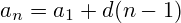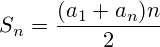# Calculator to find the nth & Sum of all terms of a Arithmetic Sequence#A Step By Step Arithmetic Progression Calculator

An online arithmetic sequence numbers calculation with step by step explanation.

Step by Step calculation

## Arithmetic Sequence Definition

definition: an = a1 + f × (n-1)
example: 1, 3, 5, 7, 9 11, 13, ...

Arithmetic progression is a sequence of numbers in which each differs from the preceding one by a constant quantity (e.g. 1, 2, 3, 4, etc.; 9, 7, 5, 3, etc.).

This constant difference is called common difference.each member of progression can be expressed asSum of the n members of arithmeic progression can be expressed as##Age Calculators ►

Horse Age Calculator , Cow Age Calculator , Cat Age Calculator , Dog Age Calculator, Human Age Calculator 100 Days Old Calculator Date Difference Calculator